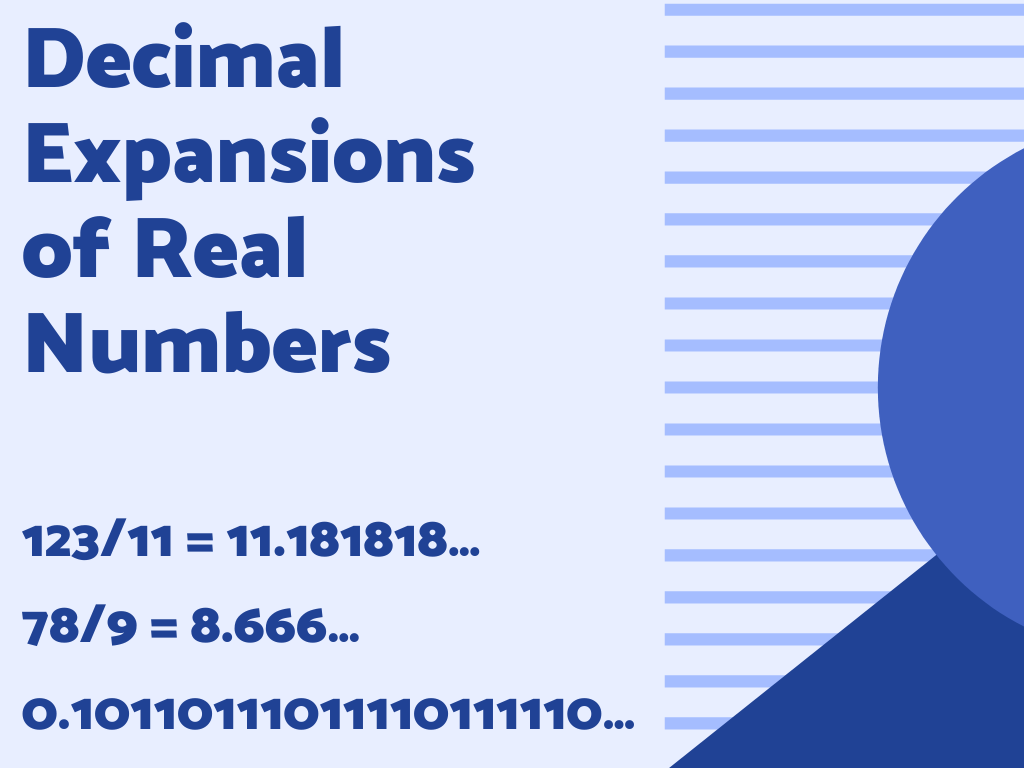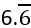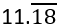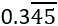# Decimal Expansions of Real Numbers Class 9th

As we know that collections of all rational numbers and irrational numbers are known as real numbers. In this section, we shall study decimal expansions of real numbers means decimal expansions of rational numbers and irrational numbers. First, we start with rational numbers.

### Decimal Expansions of Rational Numbers

Rational numbers are in the form of p/q where p and q are integers and q ≠ 0. To get the decimal expansions, we have to do the division process. Let us take some examples to understand it.

Example – Find the decimal expansions of 20/3, 9/4, and 2/7.

Solution – We shall do the division process for the decimal expansions.

In the above example, we can notice three things and they are –

(1) The remainder is either 0 after a certain stage (in 9/4) or starts repeating itself (in 20/3 and 2/7).

(2) The remainders entries which are repeating are less than the divisor.

(in 20/3, one number that is 2, is repeating itself in the remainder and that is less than the divisor 3)

(in 2/7, six entries 645132, are repeating themselves, and that are less than the divisor 7)

(3) If remainders repeat, then the digits in the quotients are also repeating.

(in 20/3, digit 6 is repeating in the quotient)

(in 2/7, digits 285714 are repeating in the quotient)

The above three things are true for all the rational numbers of the form p/q (p and q integers and q ≠ 0). If we divide p by q then two cases happen, either the remainder becomes zero or never becomes zero which means we get repeating remainders. Let us discuss each case separately.

#### Case I – When the Remainder Becomes Zero

In the division process of any rational number if we get remainder 0 after a certain stage then this type of decimal expansion is called terminating decimal expansion.

In 9/4 in the above example, we get the remainder 0 after some steps and the decimal expansion of 9/4 is 2.25. If we take other examples, like 3/2 = 1.5, 19/8 = 2.375, 567/10 = 56.7 then in all these examples, the decimal expansion terminates after a finite number of steps.

#### Case II – When the Remainder Never Becomes Zero

In the division process, we never get remainder 0 at any stage or in other words, we can say that we get repeating entries in the remainder and repeating digits in the quotient. This type of decimal expansion is called non-terminating recurring (repeating) decimal expansion.

In the above example, in 20/3 and 2/7, we get repeating entries in remainders and repeating digits in quotients. Other examples are 485/3 = 161.666…, 78/9 = 8.666…, 123/11 = 11.181818…, 258/7 = 36.857142857142… in all these examples, the decimal expansion is non-terminating recurring.

We can write repeating digits in the quotient by showing a bar line above them.

The above explanation shows that there are only two choices for decimal expansions of rational numbers either they are terminating or non-terminating recurring.

If we have given decimal expansions either terminating or non-terminating recurring in the questions and we have to prove that the decimal expansion is a rational number then we can prove this, but how? Let’s help ourselves by taking some examples.

Example (1) Show that 1.75489 is a rational number.

Solution – The decimal expansion in 1.75489 is terminating so we can easily convert this in the form of p/q.

1.75489 = 175489/100000

175489/100000 is a rational number because it is in the form of p/q where p and q are integers and q ≠ 0.                     Ans.

Example (2) Express 6.666… =in the form of p/q, where p and q are integers and q ≠ 0.

Solution – The decimal expansion in 6.666… is non-terminating recurring so we have to follow some steps to solve it.

Step I – Letbe equal to x. So,

x == 6.666…      …………. (1)

Step II – In 6.666…, only one digit is repeating just after the decimal. So, multiplying equation (1) by 10.

10x = 6.666…×10

10x = 66.666…    …………. (2)

Step III – Subtracting equation (1) from equation (2).

10x – x = 66.666… – 6.666…

9x = 60

x = 60/9

x = 20/3

x = 20/3 is in the form of p/q.            Ans.

Note – 1) When we express non-terminating recurring decimal expansion in the form of p/q, then we must have noticed one thing that, in decimal expansion, how many digits are repeating? If one digit is repeating we shall multiply by 10, if two digits are repeating we’ll multiply by 100, and so on.

2) In decimal expansion, repeating digits should start just after the decimal, if it is not, then we have to shift the decimal just before the repeating digits by multiplying by multiples of 10.

Example (3) Express 11.181818… =in the form of p/q, where p and q are integers and q ≠ 0.

Solution –

Step I – Let x = 11.181818…       …………(1)

Step II – Since two digits are repeating, so we’ll multiply equation (1) by 100.

100x = 11.181818…×100

100x = 1118.181818…        …………..(2)

Step III – Subtracting equation (1) from equation (2).

100x – x = 1118.181818… – 11.181818…

99x = 1107

x = 1107/99

x = 123/11

Therefore, the p/q form of the decimal expansion 11.181818… is 123/11.           Ans.

Example (4) Show that 0.3454545… =can be expressed in the form p/q, where p and q are integers and q ≠ 0.

Solution – Step I – Let x = 0.3454545…    ………..(1)

Step II – Since two digits are repeating but just after the decimal, digit 3 is not repeating so first, we shall shift the decimal to one place right side by multiplying equation (1) by 10.

10x = 0.3454545…×10

10x = 03.454545…      ………..(2)

Step III – Now, we’ll multiply equation (2) by 100, because two digits are repeating.

100×10x = 3.454545…×100

1000x = 345.454545…     ………….(3)

Step IV – Subtracting equation (2) from equation (3).

1000x – 10x = 345.454545… – 3.454545…

990x = 342

x = 342/990

x = 38/110

Therefore, the p/q form of 0.3454545… is 38/110.         Ans.

With the help of the above examples, we can conclude that every number with terminating or non-terminating recurring decimal expansion can be expressed in the form p/q (p and q are integers and q ≠ 0).

We can state our results in the following form:

The decimal expansion of a rational number is either terminating or non-terminating recurring and we can also say that a number whose decimal expansion is terminating or non-terminating recurring is a rational number.

### Decimal Expansions of Irrational Numbers

In the above section, we have studied that the decimal expansion of a rational number is either terminating or non-terminating recurring. On the basis of this, we can find decimal expansions of irrational numbers. We can conclude that the decimal expansions of irrational numbers are non-terminating non-recurring (non-repeating).

For irrational numbers, we can state:

The decimal expansion of an irrational number is non-terminating non-recurring and we can also say that a number whose decimal expansion is non-terminating non-recurring is an irrational number.

Examples – 1) √2 = 1.41421356237309504880…

2) √3 = 1.732050807568877…

3) 0.10110111011110111110…

4) π = 3.141592653589793238…

Note – We often use 22/7 as an approximate value for π, but the actual value of π is not equal to 22/7. Since, π = 3.141592653589793238… and 22/7 = 3.142857142857142857…

Only two digits after the decimal are same but the decimal expansions are not same. Therefore, π ≠ 22/7.

Now, let us see how to obtain irrational numbers with the help of an example.

Example – Find an irrational number between 1/3 and 2/3.

Solution – First, we’ll find decimal expansions of 1/3 and 2/3.

1/3 = 0.333…

2/3 = 0.666…

We have to find an irrational number between 0.333… and 0.666…

We’ll find a number whose decimal expansion is non-terminating non-recurring lying between them. There are infinitely many such numbers. 0.505505550… is such an irrational number that lies between 1/3 and 2/3.             Ans.

Decimal Expansions of Real Numbers Class 9th in Hindi

More About Decimal Expansions of Real Numbers

Rate this post